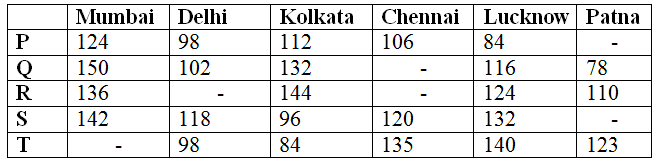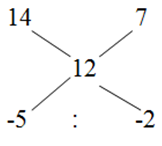# IBPS PO Mains 2018 – Quantitative Aptitude Questions Day-21

Dear Readers, Bank Exam Race for the Year 2018 is already started, To enrich your preparation here we have providing new series of Practice Questions on Quantitative Aptitude – Section. Candidates those who are preparing for IBPS PO Mains 2018 Exams can practice these questions daily and make your preparation effective.

[WpProQuiz 4214]

1) The Average marks obtained by 63 students in an examination is 12. If the average marks of passed students are 14 and that of failed students are 7, then find the number of students who passed the examination?

a) 38

b) 42

c) 45

d) 34

e) None of these

2) Two pipes can fill an empty tank in 3 hours 15 min. The pipes are opened simultaneously and it is found that due to leakage in the bottom of the tank, it takes 45 min more to fill the tank. If the tank is full, at what time would the leakage empty the full tank?

a) 16 hours 25 min

b) 15 hours 45 min

c) 17 hours 20 min

d) 18 hours 30 min

e) None of these

3) Agila, Manoj and Prithika enter into a partnership. Agila invests 2 times as much as Manoj’s investment and Manoj invests 4/7th of Prithika’s investment. If the total profit earned at the end of the year is Rs. 123500, then find the share of Manoj?

a) Rs. 28000

b) Rs. 22000

c) Rs. 26000

d) Rs. 30000

e) None of these

4) The CI on a certain sum of money for 3 years at 5 % per annum is Rs. 7881.25. Then find the principle?

a) Rs. 45000

b) Rs. 50000

c) Rs. 42000

d) Rs. 36000

e) None of these

5) Two stations A and B are 180 km apart on a straight line. One train starts from A at 8 a.m. and travels towards B at 30 km/hr. Another train starts from B at 9 a.m. and travels towards A at a speed of 45 km/hr. At what time will they meet?

a) 10 a.m.

b) 11 a.m.

c) 11.30 a.m.

d) 12 a.m.

e) None of these

Directions (Q. 6 – 10) Study the following information carefully and answer the given questions:

The table shows the total sales value (in lakhs) of five famous books P, Q, R, S and T in six different cities. Some values are missing.6) If the average sales value for all the given famous books in Mumbai is 136 lakhs, then find the ratio of total sales value of book T in Mumbai to that of total sales value of for all the given famous books together in Kolkata?

a) 25 : 68

b) 16 : 71

c) 32 : 93

d) 45 : 121

e) None of these

7) If the average sales value of the book R for all the given cities together is 130 lakhs and the total sales value of book R in Delhi is 10 lakhs less than the total sales value of book R in Chennai, then the total sales value of book R in Delhi is approximately what percentage less than the total sales value of book R in Chennai?

a) 7 %

b) 15 %

c) 22 %

d) 28 %

e) 35 %

8) If total sales value of all the given books in Patna is 547 lakhs and the total sales value of book S in Patna is 32 lakhs more than the total sales value of book P in Patna, then find the difference between the total sales value of book P to that of book S in all the given cities together?

a) 134 lakhs

b) 92 lakhs

c) 116 lakhs

d) 122 lakhs

e) None of these

9) The total sales value in Kolkata is approximately what percentage more/less than the total sales value in Lucknow of all the given famous books together?

a) 15 % more

b) 5 % less

c) 20 % less

d) 15 % less

e) 5 % more

10) The average sales values of book Q, R and S together in Delhi is 116 lakhs, then find the total sales value of book R in Delhi?

a) 128 lakhs

b) 110 lakhs

c) 86 lakhs

d) 72 lakhs

e) None of these

Let the number of passed students be x,

63*12 = 14x + (63 – x)*7

756 = 14x + 441 – 7x

756 – 441 = 7x

7x = 315

Total number of passed students (x) = 45

Shortcut:= > 5 : 2

7’s = 63

1’s = 9

Total number of passed students = 5’s = 45

Two pipes can fill an empty tank in = 3 hours 15 min = 3 15/60 = 3 1/4

But due to the leakage, it takes 45 min more,

So, Total time taken to fill the tank = 4 hours

Required number of hours = 4/13 – (1/4) = (16 – 13)/52 = 3/52

The leakage empty the full tank in 52/3 = 17 hours 20 min

The investment of Agila, Manoj = 2 : 1

Agila’s investment = (4/7)* Prithika’s investment

The investment of Manoj and Prithika = 4 : 7

The investment of Agila, Manoj and Prithika = 8 : 4 : 7

Here, the period is not given. So,

The ratio of investment = The ratio of profit

Total profit = Rs. 123500

19’s = 123500

1’s = 6500

The share of Manoj = 4’s = Rs. 26000

C.I = P*[(1 + (r/100))2 – 1]

7881.25 = P*[(1 + 5/100)3 – 1]

7881.25 = P*[(105/100)3 – 1]

7881.25 = P*[(21/20)3 – 1]

7881.25 = P*[(9261/8000) – 1]

7881.25 = P*(1261/8000)

7881.25*(8000/1261) = P

P = Rs. 50000

They meet x hours after 8 a.m.

Distance covered by A in x hours = 30x km

Distance covered by B in (x – 1) hours = 45(x – 1) km

30x + 45(x – 1) = 180

30x + 45x = 180 + 45

75x = 225

X = 3

They meet at 11 a.m.

Direction (6-10) :

The average sales value for all the given famous books in Mumbai = 136 lakhs

The total sales value for all the given famous books in Mumbai = 136*5 = 680 lakhs

Total sales value of book T in Mumbai

= > 680 – (124 + 150 + 136 + 142) = 128 lakhs

The total sales value of for all the given famous books together in Kolkata

= > 112 + 132 + 144 + 96 + 84 = 568 lakhs

Required ratio = 128 : 568 = 16 : 71

The average sales value of the book R for all the given cities together = 130 lakhs

Total sales value of the book R for all the given cities together = 130*6 = 780 lakhs

Total sales value of book R in Delhi = Total sales value of book R in Chennai – 10 lakhs

C – D = 10 — > (1)

Total sales value of book R in Delhi and Chennai

= > 780 – (136 + 144 + 124 + 110)

= > 266 lakhs

C + D = 266 —-> (2)

By solving the equation (1) and (2), we get,

Total sales value of book R in Chennai = 138 lakhs

Total sales value of book R in Delhi = 128 lakhs

Required % = [(138 – 128)/138]*100 = 7 %

Total sales value of all the given books in Patna = 547 lakhs

The total sales value of book P and S in Patna

= > 547 – (78 + 110 + 123)

= > 236 lakhs

S + P = 236 –à (1)

The total sales value of book S in Patna = 32 lakhs + The total sales value of book P in Patna

S – P = 32 –à (2)

By solving the equation (1) and (2),

The total sales value of book S in Patna = 134 lakhs

The total sales value of book P in Patna = 102 lakhs

The total sales value of book P in all the given cities together

= > (124 + 98 + 112 + 106 + 84 + 102) = 626 lakhs

The total sales value of book S in all the given cities together

= > (142 + 118 + 96 + 120 + 132 + 134) = 742 lakhs

Required difference = 742 – 626 = 116 lakhs

The total sales value in Kolkata of all the given famous books together

= > 112 + 132 + 144 + 96 + 84 = 568 lakhs

The total sales value in Lucknow of all the given famous books together

= > 84 + 116 + 124 + 132 + 140 = 596 lakhs

Required % = [(596 – 568)/596]*100

= > 5 % less

The average sales values of book Q, R and S together in Delhi = 116 lakhs

The total sales values of book Q, R and S together in Delhi = 116*3 = 348 lakhs

The total sales value of book R in Delhi = 348 – (102 + 118) = 128 lakhs

Daily Practice Test Schedule | Good Luck

 Topic Daily Publishing Time Daily News Papers & Editorials 8.00 AM Current Affairs Quiz 9.00 AM Current Affairs Quiz (Hindi) 9.30 AM IBPS Clerk Prelims – Reasoning 10.00 AM IBPS Clerk Prelims – Reasoning (Hindi) 10.30 AM IBPS Clerk Prelims – Quantitative Aptitude 11.00 AM IBPS Clerk Prelims – Quantitative Aptitude (Hindi) 11.30 AM Vocabulary (Based on The Hindu) 12.00 PM IBPS PO Prelims – English Language 1.00 PM SSC Practice Questions (Reasoning/Quantitative aptitude) 2.00 PM IBPS PO/Clerk – GK Questions 3.00 PM SSC Practice Questions (English/General Knowledge) 4.00 PM Daily Current Affairs Updates 5.00 PM IBPS PO Mains – Reasoning 6.00 PM IBPS PO Mains – Quantitative Aptitude 7.00 PM IBPS PO Mains – English Language 8.00 PM